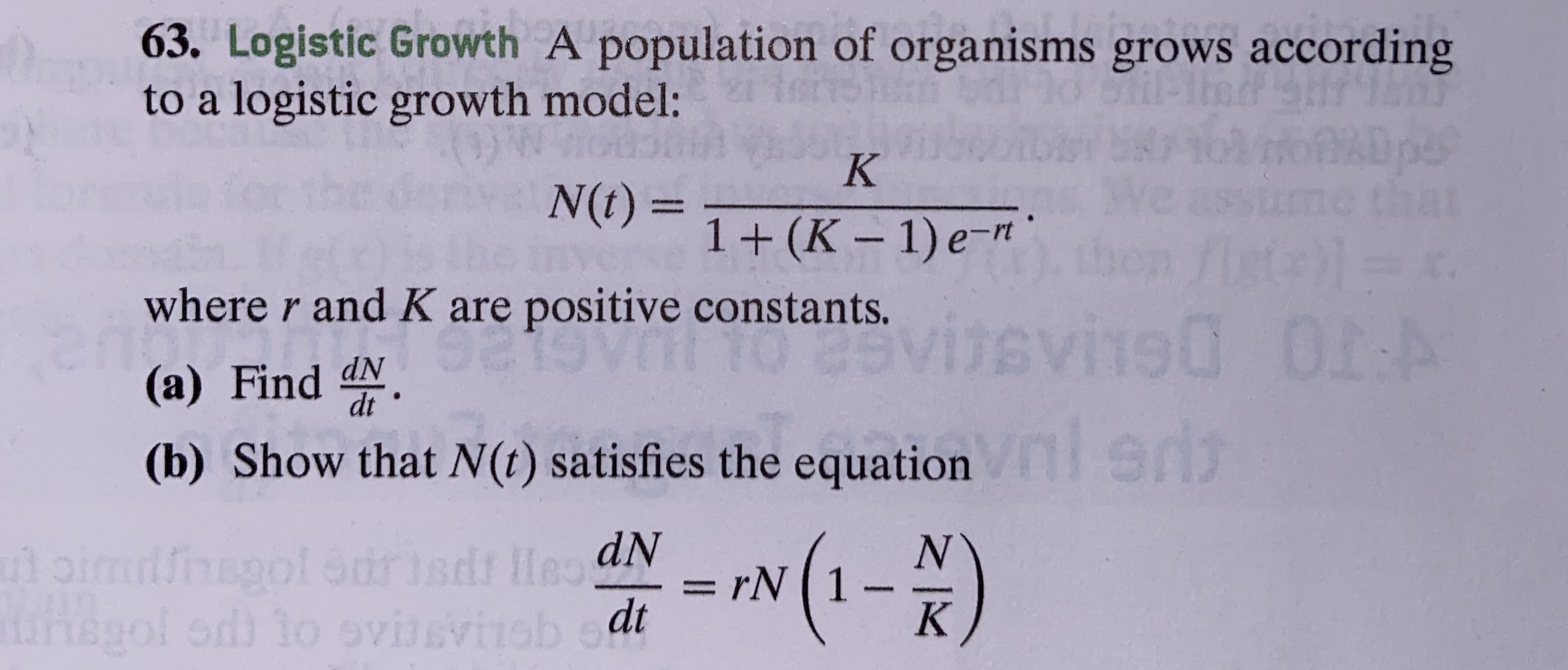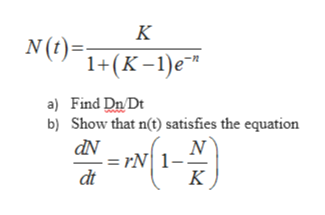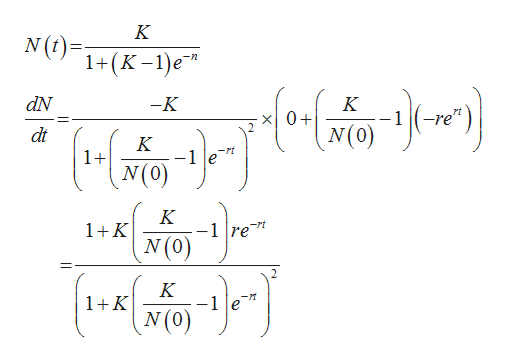# 63. Logistic Growth A population of organisms grows accordingto a logistic growth model:KN(t)=1+ (K-1) e-mtwhere r and K are positive constants.0I:Avisvi90(a) Find dN.ST(b) Show that N(t) satisfies the equation1-)NrN 1Kutoimiiol Strisdr HeaNgol od to ovissvisb et

Questionhelp_outlineImage Transcriptionclose63. Logistic Growth A population of organisms grows according to a logistic growth model: K N(t)= 1+ (K-1) e-mt where r and K are positive constants. 0I:A visvi90 (a) Find dN. ST (b) Show that N(t) satisfies the equation 1-) N rN 1 K utoimiiol Strisdr HeaN gol od to ovissvisb et fullscreen
check_circleExpert Solution
Step 1

A population of organisms grow according to a logistic growth modelhelp_outlineImage TranscriptioncloseК N(t)= 1+(K-1)e a) Find Dn Dt b) Show that n(t) satisfies the equation N = rN 1- К dt fullscreen
Step 2

Find the first order deriva...help_outlineImage TranscriptioncloseK N(t) 1+(K -1)e dN -K K x0+ N(0) -re" dt K -rt 1+ K -1 re" N(0) 1K K 1K fullscreen

### Want to see the full answer?

See Solution

#### Want to see this answer and more?

Solutions are written by subject experts who are available 24/7. Questions are typically answered within 1 hour*

See Solution
*Response times may vary by subject and question
Tagged in

### Other